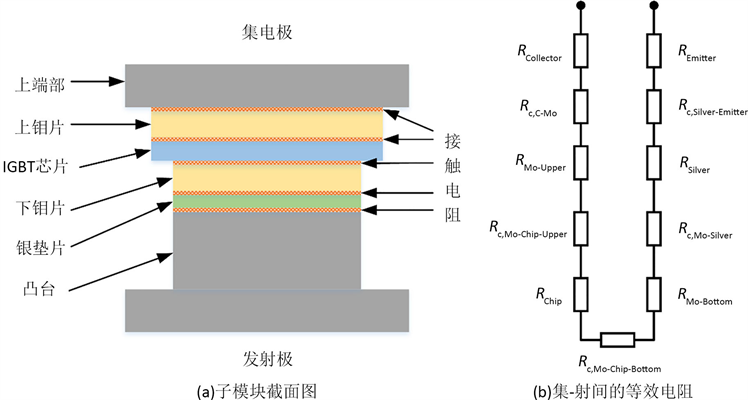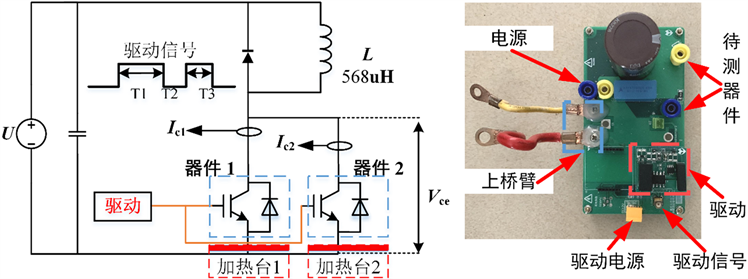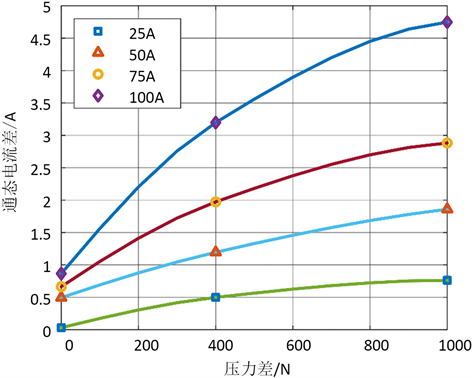﻿ 多芯片并联压接式IGBT中压力不均对电流分布的影响分析

# 多芯片并联压接式IGBT中压力不均对电流分布的影响分析Influence of Uneven Pressure Distribution on Current Distribution in Paralleled Multi-Chips Press Pack IGBT

Abstract: As a core component of the flexible HVDC equipment, the press pack IGBT device has uneven pressure distribution within the device due to factors such as manufacturing process and device aging at a long-time operation, which results in uneven current distribution among the paralleled sub-modules. This reduces the reliability of some sub-modules and accelerates them aging speed. In order to study the relationship between the pressure difference in the press pack IGBT and the degree of current imbalance, this paper analyzes the reason for the uneven current of the paralleled modules caused by the uneven pressure distribution. From the experiment under different pressure differences, data of current distribution data was collected. The relationship between the difference in pressure and the difference in current distribution is clarified through the analysis of the experimental results, which provides a reference for design optimization and exploring the evolution of aging failure in press pack IGBTs.

Abstract:

1. 引言

2. 压力不均导致不均流的原因分析Figure 1. Cross section of press-pack IGBT and its equivalent on-resistance

${R}_{\text{ECC}}=\frac{{\rho }_{1}+{\rho }_{2}}{2}\sqrt{\frac{\pi H}{F}}$ (1)

$\begin{array}{l}{R}_{\text{ECC,total}}={R}_{\text{c,C-Mo}}+{R}_{\text{c,Mo-Chip-Upper}}+{R}_{\text{c,Mo-Chip-Bottom}}+{R}_{\text{c,Mo-Silver}}+{R}_{\text{c,Silver-Emmiter}}\\ {R}_{\text{Layers}}={R}_{\text{Collector}}+{R}_{\text{Mo-Upper}}+{R}_{\text{Chip}}+{R}_{\text{Mo-Bottom}}+{R}_{\text{Silver}}+{R}_{\text{Emitter}}\end{array}$ (2)

${R}_{\text{CE}}={R}_{\text{ECC,total}}+{R}_{\text{Layers}}$ (3)(4)

$\Delta I=\frac{|{R}_{\text{ECC,total1}}\left({F}_{1}\right)-{R}_{\text{ECC,total2}}\left({F}_{2}\right)|}{{R}_{\text{ECC,total1}}\left({F}_{1}\right)+{R}_{\text{Layers1}}+{R}_{\text{ECC,total1}}\left({F}_{2}\right)+{R}_{\text{Layers1}}}\cdot {I}_{\text{C}}$ (5)

3. 不同压力差异下的不均流程度实验及分析Figure 2. Schematic diagram of the equivalent experiment circuit and board for test

3.1. 压力差异对不均流程度的影响Table 1. Current imbalance under different pressureFigure 3. Current imbalance under different pressure difference

3.2. 温度差异对压力造成的不均流程度的影响Table 2. Relative change of current imbalance in different pressure distribution

4. 总结

1) 压力的不均通过改变压接式IGBT的接触面粗糙度，导致器件内部不同模块的接触电阻产生差异，进而导致器件内部产生的不均流现象。

2) 当选择小压力值最小的子模块作为参照时，内部并联子模块间的压力差异越大，模块间的不均流程度越严重，电流差异随压力差异表现为指数关系；并且随着压力差异的增大，电流差异的相对增量逐渐减小。压力参考值为1200 N时，压力差异绝对值在参考值两侧变化对不均流程度的影响效果不同。

3) 不同的压力分布情况下，不同的压力差异造成的不均流程度可能相似；即在分析压力对电流分布的影响时，需要固定压力的参考点，不可仅凭压力大小差异来判断或估计压接式IGBT器件中的不均流程度。

4) 在一定温度范围内，压接式IGBT内部温度分布的变化，不会造成由压力差异导致的不均流程度发生明显变化。

 段雯娟. 中美新气候行动令人鼓舞[J]. 地球, 2015(11): 44-46.

 胡航海, 李敬如, 杨卫红, 李红军. 柔性直流输电技术的发展与展望[J]. 电力建设, 2011, 32(5): 62-66.

 中国能源研究会. 中国能源发展报告2018 [J]. 中国电业, 2018(10): 2.

 Mo, S., Zhao, Z., Sun, P., Lu, Y. and Tang, X. (2017) Influence of Package Parasitic Inductance on Transient Current Distribution Characteristics of Press Pack Igbt. 2017 6th Asia-Pacific Conference on Antennas and Propagation (APCAP), Xi’an, 16-19 October 2017, 1-3.
https://doi.org/10.1109/APCAP.2017.8420381

 顾妙松, 崔翔, 彭程, 唐新灵,杨 艺烜, 李学宝, 赵志斌. 外部汇流母排对压接型IGBT器件内部多芯片并联均流特性的影响[J]. 中国电机工程学报, 2020, 40(1): 234-245+390.

 Wei, L., Li, H., Chen, M., et al. (2019) Investigation on the Effects of Unbalanced Clamping Force on Multi-chip Press Pack IGBT Modules. IEEE Journal of Emerging & Selected Topics in Power Electronics, 7, 2314-2322. |
https://doi.org/10.1109/JESTPE.2018.2876768

 Poller, T., Lutz, J., D’Arco, S., et al. (2013) Determination of the Thermal and Electrical Contact Resistance in Press-Pack IGBTs. 2013 IEEE 15th European Conference on Power Electronics and Applications (EPE), Lille, 2-6 September 2013, 1-9.
https://doi.org/10.1109/EPE.2013.6634440

 Ortiz Gonzalez, J., Alatise, O., Aliyu, A., et al. (2017) Evaluation of SiC Schottky Diodes Using Pressure Contacts. IEEE Transactions on Industrial Electronics, 64, 8213-8223.
https://doi.org/10.1109/TIE.2017.2677348

 Rajaguru, P., Lu, H., Bailey, C., et al. (2015) Electro-Thermo-Mechanical Modelling and Analysis of the Press Pack Diode in Power Electronics. IEEE International Workshop on Thermal Investigations of Ics & Systems, Paris, 30 September-2 October 2015, 1-6.
https://doi.org/10.1109/THERMINIC.2015.7389607

Top